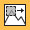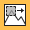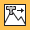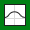Main Content

## Deep Learning Network Composition

To create a custom layer that itself defines a layer graph, you can specify a `dlnetwork` object as a learnable parameter. This method is known as network composition. You can use network composition to:

• Create a single custom layer that represents a block of learnable layers, for example, a residual block.

• Create a network with control flow, for example, a network with a section that can dynamically change depending on the input data.

• Create a network with loops, for example, a network with sections that feed the output back into itself.

For an example showing how to define a custom layer containing a learnable `dlnetwork` object, see Define Nested Deep Learning Layer.

For an example showing how to train a network with nested layers, see Train Deep Learning Network with Nested Layers.

### Automatically Initialize Learnable `dlnetwork` Objects for Training

You can create a custom layer and allow the software to automatically initialize the learnable parameters of any nested `dlnetwork` objects after the parent network is fully constructed. Automatic initialization of the nested network means that you do not need to keep track of the size and shape of the inputs passed to each custom layer containing a nested `dlnetwork`

To take advantage of automatic initialization, you must specify that the constructor function creates an uninitialized `dlnetwork` object. To create an uninitialized `dlnetwork` object, set the `Initialize` name-value option to false. You do not need to specify an input layer, so you do not need to specify an input size for the layer.

```function layer = myLayer % Initialize layer properties. ... % Define network. layers = [ % Network layers go here. ]; layer.Network = dlnetwork(lgraph,'Initialize',false); end```

When the parent network is initialized, the learnable parameters of any nested `dlnetwork` objects are initialized at the same time. The size of the learnable parameters depends on the size of the input data of the custom layer. The software propagates the data through the nested network and automatically initializes the parameters according to the propagated sizes and the initialization properties of the layers of the nested network.

If the parent network is trained using the `trainNetwork` function, then any nested `dlnetwork` objects are initialized when you call `trainNetwork`. If the parent network is a `dlnetwork`, then any nested `dlnetwork` objects are initialized when the parent network is constructed (if the parent `dlnetwork` is initialized at construction) or when you use the `initialize` function with the parent network (if the parent `dlnetwork` is not initialized at construction).

Alternatively, instead of deferring initialization of the nested network, you can construct the custom layer with the nested network already initialized. This means that the nested network is initialized before the parent network This requires manually specifying the size of any inputs to the nested network. You can do so either by using input layers or by providing example inputs to the `dlnetwork` constructor function. Because you must specify the sizes of any inputs to the `dlnetwork` object, you might need to specify input sizes when you create the layer. For help determining the size of the inputs to the layer, you can use the `analyzeNetwork` function and check the size of the activations of the previous layers.

### Predict and Forward Functions

Some layers behave differently during training and during prediction. For example, a dropout layer performs dropout only during training and has no effect during prediction. A layer uses one of two functions to perform a forward pass: `predict` or `forward`. If the forward pass is at prediction time, then the layer uses the `predict` function. If the forward pass is at training time, then the layer uses the `forward` function. If you do not require two different functions for prediction time and training time, then you can omit the `forward` function. In this case, the layer uses `predict` at training time.

When implementing the `predict` and the `forward` functions of the custom layer, to ensure that the layers in the `dlnetwork` object behave in the correct way, use the `predict` and `forward` functions for `dlnetwork` objects, respectively.

Custom layers with learnable `dlnetwork` objects do not support custom backward functions.

You must still assign a value to the memory output argument of the `forward` function.

This example code shows how to use the `predict` and `forward` functions with `dlnetwork` input.

```function Z = predict(layer,X) % Convert input data to formatted dlarray. X = dlarray(X,'SSCB'); % Predict using network. dlnet = layer.Network; Z = predict(dlnet,X); % Strip dimension labels. Z = stripdims(Z); end function [Z,memory] = forward(layer,X) % Convert input data to formatted dlarray. X = dlarray(X,'SSCB'); % Forward pass using network. dlnet = layer.Network; Z = forward(dlnet,X); % Strip dimension labels. Z = stripdims(Z); memory = []; end```

If the `dlnetwork` object does not behave differently during training and prediction, then you can omit the forward function. In this case, the software uses the `predict` function during training.

### Supported Layers

Custom layers support `dlnetwork` objects that do not require state updates. This means that the `dlnetwork` object must not contain layers that have a state, for example, batch normalization and LSTM layers.

This list shows the built-in layers that fully support network composition.

#### Input Layers

LayerDescription`imageInputLayer`

An image input layer inputs 2-D images to a network and applies data normalization.`image3dInputLayer`

A 3-D image input layer inputs 3-D images or volumes to a network and applies data normalization.`sequenceInputLayer`

A sequence input layer inputs sequence data to a network.`featureInputLayer`

A feature input layer inputs feature data to a network and applies data normalization. Use this layer when you have a data set of numeric scalars representing features (data without spatial or time dimensions).

#### Convolution and Fully Connected Layers

LayerDescription`convolution2dLayer`

A 2-D convolutional layer applies sliding convolutional filters to the input.`convolution3dLayer`

A 3-D convolutional layer applies sliding cuboidal convolution filters to three-dimensional input.`groupedConvolution2dLayer`

A 2-D grouped convolutional layer separates the input channels into groups and applies sliding convolutional filters. Use grouped convolutional layers for channel-wise separable (also known as depth-wise separable) convolution.`transposedConv2dLayer`

A transposed 2-D convolution layer upsamples feature maps.`transposedConv3dLayer`

A transposed 3-D convolution layer upsamples three-dimensional feature maps.`fullyConnectedLayer`

A fully connected layer multiplies the input by a weight matrix and then adds a bias vector.

#### Activation Layers

LayerDescription`reluLayer`

A ReLU layer performs a threshold operation to each element of the input, where any value less than zero is set to zero.`leakyReluLayer`

A leaky ReLU layer performs a threshold operation, where any input value less than zero is multiplied by a fixed scalar.`clippedReluLayer`

A clipped ReLU layer performs a threshold operation, where any input value less than zero is set to zero and any value above the clipping ceiling is set to that clipping ceiling.`eluLayer`

An ELU activation layer performs the identity operation on positive inputs and an exponential nonlinearity on negative inputs.`swishLayer`

A swish activation layer applies the swish function on the layer inputs.`tanhLayer`

A hyperbolic tangent (tanh) activation layer applies the tanh function on the layer inputs.`softmaxLayer`

A softmax layer applies a softmax function to the input.

#### Normalization, Dropout, and Cropping Layers

LayerDescription`groupNormalizationLayer`

A group normalization layer normalizes a mini-batch of data across grouped subsets of channels for each observation independently. To speed up training of the convolutional neural network and reduce the sensitivity to network initialization, use group normalization layers between convolutional layers and nonlinearities, such as ReLU layers.`layerNormalizationLayer`

A layer normalization layer normalizes a mini-batch of data across all channels for each observation independently. To speed up training of recurrent and multi-layer perceptron neural networks and reduce the sensitivity to network initialization, use layer normalization layers after the learnable layers, such as LSTM and fully connected layers.`crossChannelNormalizationLayer`

A channel-wise local response (cross-channel) normalization layer carries out channel-wise normalization.`dropoutLayer`

A dropout layer randomly sets input elements to zero with a given probability.`crop2dLayer`

A 2-D crop layer applies 2-D cropping to the input.

#### Pooling and Unpooling Layers

LayerDescription`averagePooling2dLayer`

An average pooling layer performs downsampling by dividing the input into rectangular pooling regions and computing the average values of each region.`averagePooling3dLayer`

A 3-D average pooling layer performs downsampling by dividing three-dimensional input into cuboidal pooling regions and computing the average values of each region.`globalAveragePooling2dLayer`

A global average pooling layer performs downsampling by computing the mean of the height and width dimensions of the input.`globalAveragePooling3dLayer`

A 3-D global average pooling layer performs downsampling by computing the mean of the height, width, and depth dimensions of the input.`maxPooling2dLayer`

A max pooling layer performs downsampling by dividing the input into rectangular pooling regions, and computing the maximum of each region.`maxPooling3dLayer`

A 3-D max pooling layer performs downsampling by dividing three-dimensional input into cuboidal pooling regions, and computing the maximum of each region.`globalMaxPooling2dLayer`

A global max pooling layer performs downsampling by computing the maximum of the height and width dimensions of the input.`globalMaxPooling3dLayer`

A 3-D global max pooling layer performs downsampling by computing the maximum of the height, width, and depth dimensions of the input.`maxUnpooling2dLayer`

A max unpooling layer unpools the output of a max pooling layer.

#### Combination Layers

LayerDescription`additionLayer`

An addition layer adds inputs from multiple neural network layers element-wise.`multiplicationLayer`

A multiplication layer multiplies inputs from multiple neural network layers element-wise.`depthConcatenationLayer`

A depth concatenation layer takes inputs that have the same height and width and concatenates them along the third dimension (the channel dimension).`concatenationLayer`

A concatenation layer takes inputs and concatenates them along a specified dimension. The inputs must have the same size in all dimensions except the concatenation dimension.

### GPU Compatibility

If the layer forward functions fully support `dlarray` objects, then the layer is GPU compatible. Otherwise, to be GPU compatible, the layer functions must support inputs and return outputs of type `gpuArray` (Parallel Computing Toolbox).

Many MATLAB® built-in functions support `gpuArray` (Parallel Computing Toolbox) and `dlarray` input arguments. For a list of functions that support `dlarray` objects, see List of Functions with dlarray Support. For a list of functions that execute on a GPU, see Run MATLAB Functions on a GPU (Parallel Computing Toolbox). To use a GPU for deep learning, you must also have a supported GPU device. For information on supported devices, see GPU Support by Release (Parallel Computing Toolbox). For more information on working with GPUs in MATLAB, see GPU Computing in MATLAB (Parallel Computing Toolbox).

Download ebook